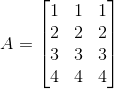Construct a 4 × 3 matrix A = [ai j] whose elements ai j are given by ai j = i

Asked by Aaryan | 1 year ago |  168

##### Solution :-

Given ai j = i

Let A = [ai j]4×3

So, the elements in a 4 × 3 matrix are

a11, a12, a13, a21, a22, a23, a31, a32, a33, a41, a42, a43

a11 = 1

a12 = 1

a13 = 1

a21 = 2

a22 = 2

a23 = 2

a31 = 3

a32 = 3

a33 = 3

a41 = 4

a42 = 4

a43 = 4

Substituting these values in matrix A we get,Answered by Sakshi | 1 year ago

### Related Questions

#### Find the values of a, b, c and d from the equations

Find the values of a, b, c and d from the equations:

#### Find x, y, a and b if

Find x, y, a and b if

#### Find x, y, a and b if

Find x, y, a and b if

Construct a 4 × 3 matrix A = [ai j] whose elements ai j are given by $$a_{i j} = \dfrac{(i – j)}{(i + j)}$$
Construct a 4 × 3 matrix A = [ai j] whose elements ai j are given by ai j = 2i + $$\dfrac{i}{j}$$